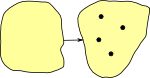# Maths - Category Theory - Initial Object

An initial object has a unique morphism to every other object in the category.

#### Initial Object in Set

Although category theory is about the external properties of objects, to get an intuitive understanding, it can be helpful to peek inside them. In set-like categories the initial object tends to be like the empty set.

In set, maps exist from any n-element to an m-element set (provided m≠0).

So every set has maps to every non-empty set, however only maps from the 0-element set are unique.

We can think of maps from the empty set as a special case of injective maps.

### Strict Initial Object

A strict initial object has no morphisms into it (apart from its own identity).

In type theory this could be related to the empty type and way to represent 'false'.

An example of a strict initial object is the empty set.

## Examples in Various Categories

Initial ObjectNotation 0
generalisation a kind of colimit
universal cone over diagram
examples: set:

Ø = {}

empty set

group (null object) trivial group (just identity element)
topological space empty space
poset least element (if exists)
monoid trivial monoid
semigroup empty semigroup
Rng ring of integers Z
fields does not have initial object
Vec zero object
Top empty space
Grf the null graph (without vertices and edges)
Ω-Alg
algebra with signature Ω
initial (term) algebra whose carrier consists of all finite trees.
Cat empty category